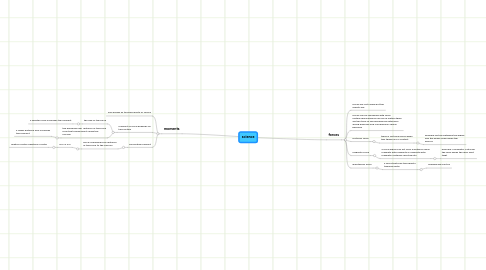# science

Get Started. It's Freescience## 1. forces

### 1.3. frictional force

1.3.1. there is frictional force when two things are in contact

1.3.1.1. example:friction between the wheel and the brake slows down the bicycle

### 1.4. magnetic force

1.4.1. a force which can act from a distance using magnets with magnets or magnets with magnetic materials like steel etc

1.4.1.1. example: a magnetic catch for the door keeps the door shut tight

### 1.5. gravitaional force

1.5.1. a force that pulls the objects towards earth

1.5.1.1. example:we cant fly

## 2. moments

### 2.2. moment of force depends on two factors

2.2.1. the size of the force

2.2.1.1. a greater force increases the moment

2.2.2. the perpendicular distance of the force from the turning point called the fulcrum

2.2.2.1. a larger distance also increases the moment

### 2.3. calculating moment

2.3.1. force x perpendicular distance of the force to the fulcrum

2.3.1.1. NM=n x m

2.3.1.1.1. newton-metre=newtons x metre